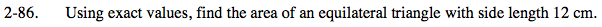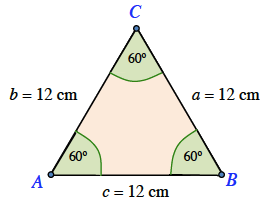### Home > PC > Chapter 2 > Lesson 2.3.2 > Problem2-86

2-86.

Using exact values, find the area of an equilateral triangle with side length 12 cm. Homework Help ✎Sketch an equilateral triangle. Label the angles and sides.

Drop a perpendicular height from C to the base AB.

What kind of a triangle is ΔCDB?

How long is DB?

Using the Pythagorean Theorem, find the height 'h'.30° 60° 90° triangle

6 cm.

Finally, find the area of the triangle multiplying half the height times the base.